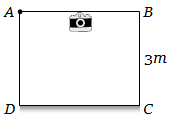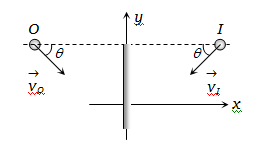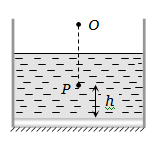Figure shows a cubical room ABCD with the wall CD as a plane mirror. Each side of the room is 3m. We place a camera at the midpoint of the wall AB. At what distance should the camera be focussed to photograph an object placed at A(a) 1.5 m                                     (b) 3 m

(c) 6 m                                         (d) More than 6 m

Concept Questions :-

Reflection at plane surface
High Yielding Test Series + Question Bank - NEET 2020

Difficulty Level:

If an object moves towards a plane mirror with a speed v at an angle $\theta$ to the perpendicular to the plane of the mirror, find the relative velocity between the object and the image(a) v                                            (b) 2v

(c) 2v cos $\theta$                                    (d) 2v sin $\theta$

Concept Questions :-

Reflection at plane surface
High Yielding Test Series + Question Bank - NEET 2020

Difficulty Level:

A plane mirror is placed at the bottom of the tank containing a liquid of refractive index $\mu$. P is a small object at a height h above the mirror. An observer vertically above P outside the liquid sees P and its image in the mirror. The apparent distance between these two will be-1. $2\mu h$

2.  $\frac{2h}{\mu }$

3. $\frac{2h}{\mu -1}$

4.  $h\left(1+\frac{1}{\mu }\right)$

Concept Questions :-

Refraction at plane surface
High Yielding Test Series + Question Bank - NEET 2020

Difficulty Level:

A thin prism having refracting angle ${10}^{°}$ is made of glass of refractive index 1.42. This prism is combined with another thin prism of glass of refractive index 1.7. This combination produces dispersion without deviation. The refracting angle of second prism should be

(a)${4}^{°}$

(b)${6}^{°}$

(c)${8}^{°}$

(d)${10}^{°}$

Concept Questions :-

Prisms
High Yielding Test Series + Question Bank - NEET 2020

Difficulty Level:

A beam of light from a source L is incident normally on a plane mirror fixed at a certain distance x from the source. The beam is reflected back as a spot on a scale placed just above the source L. When the mirror is rotated through a small angle $\theta$, the spot of light is found to move through a distance y on the scale. The angle $\theta$ is given by

(a) $\frac{y}{2x}$

(b) $\frac{y}{x}$

(c) $\frac{x}{2y}$

(d) $\frac{x}{y}$

Concept Questions :-

Reflection at plane surface
High Yielding Test Series + Question Bank - NEET 2020

Difficulty Level:

The ratio of resolving powers of an optical microscope for two wavelengths  is

(a) 8:27

(b) 9:4

(c) 3:2

(d) 16:81

Concept Questions :-

Resolution of Optical devices
High Yielding Test Series + Question Bank - NEET 2020

Difficulty Level:

Two identical glass $\left({\mu }_{g}=3/2\right)$ equi-convex lenses of focal length $f$ each are kept in contact. The space between the two lenses is filled with water $\left({\mu }_{w}=4/3\right)$. The focal length of the combination is

(a)  $f/3$             (b) $f$

(c) $\frac{4f}{3}$               (d) $\frac{3f}{4}$

Concept Questions :-

Lenses
High Yielding Test Series + Question Bank - NEET 2020

Difficulty Level:

An air bubble in a glass slab with refractive index 1.5 (near normal incidence) is 5 cm deep when viewed from one surface and  3 cm deep when viewed from the opposite face. The thickness (in cm) of the slab is

(a) 8           (b) 10

(c) 12         (d) 16

Concept Questions :-

Refraction at plane surface
High Yielding Test Series + Question Bank - NEET 2020

Difficulty Level:

A person can see clearly objects only when they lie between 50 cm and 400 cm from his eyes. In order to increase the maximum distance of distinct vision to infinity, the type and power of the correcting lens, the person has to use will be-

1. convex, +2.25 diopter

2. concave, - 0.25 diopter

3. concave, - 0.2 diopter

4. convex, + 0.15 diopter

Concept Questions :-

Human eye
High Yielding Test Series + Question Bank - NEET 2020

Difficulty Level:

An astronomical telescope has an objective and eyepiece of focal lengths 40 cm and 4 cm respectively. To view an object 200 cm away from the objective, the lenses must be separated by a distance of :
(a) 46.0 cm (b) 50.0 cm
(c) 54.0 cm (d) 37.3 cm

Concept Questions :-

Telescope